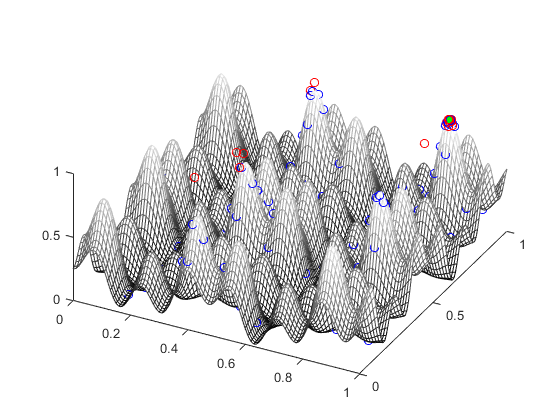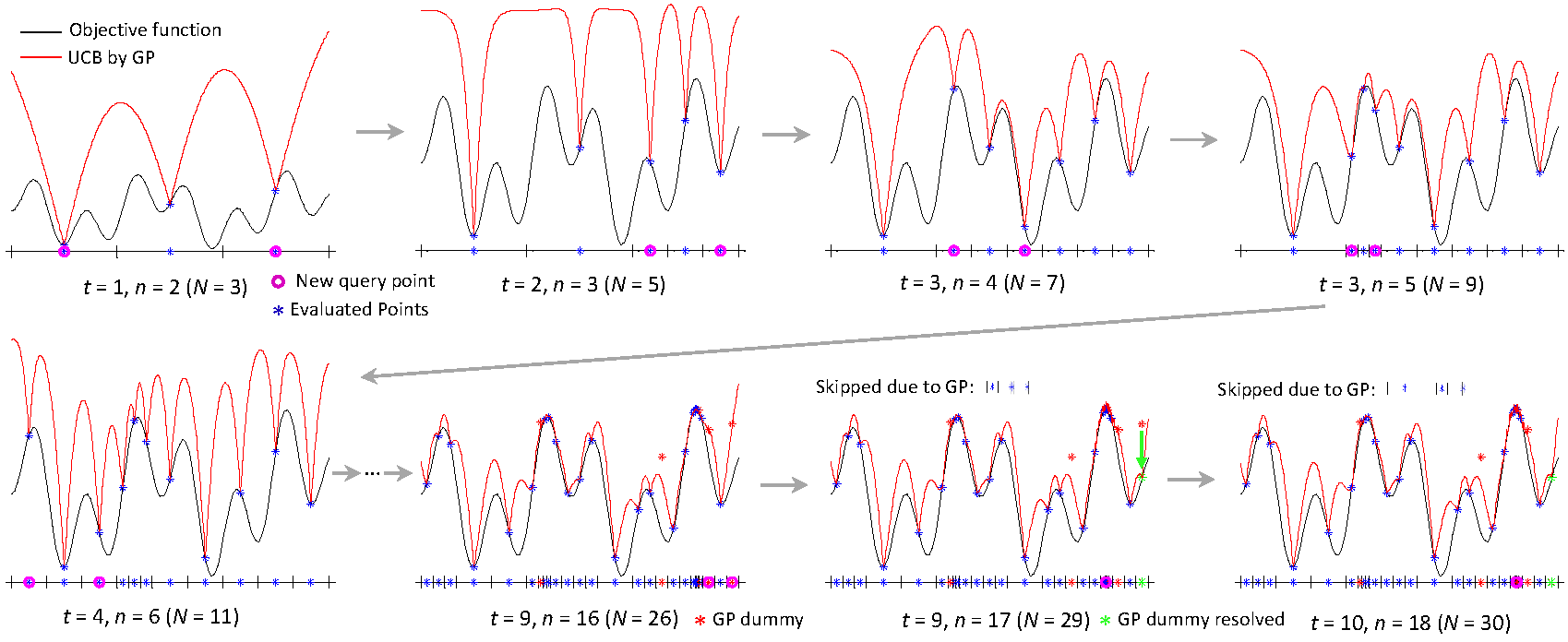## MATLAB code implementation of Bayesian optimization with exponential convergence

Main Input: a non-convex black-box deterministic function
Main output: an estimate of global optima
The form of the input function need not be known (black box) and thus a user can pass a function that simply calls, for example, a simulator as the input function.This MATLAB code implements the method that is presented in:
K. Kawaguchi, L. P. Kaelbling, T. Lozano-Pérez. Bayesian Optimization with Exponential Convergence.
In Advances in Neural Information Processing (NIPS), 2015.

The abstract of the paper that presented the method:

This paper presents a Bayesian optimization method with exponential convergence
without the need of auxiliary optimization and without the δ-cover sampling.
Most Bayesian optimization methods require auxiliary optimization: an additional
non-convex global optimization problem, which can be time-consuming
and hard to implement in practice. Also, the existing Bayesian optimization